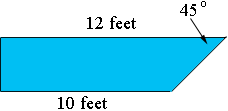SEARCH HOMEMath Central Quandaries & QueriesQuestion from Jim, a student: "The end of a swimming pool is a rectangle inclined 45° to the horizontal. The edge at the surface is 12 feet long and the submerged edge is 10 feet long. Could you draw a diagram for me ? I don't understand the Problem. ThanksHi Jim,

I'm not sure I understand either but here is a guess. I am going to assume that the other end of the pool is vertical. In this case a cross-section would look likePennyMath Central is supported by the University of Regina and The Pacific Institute for the Mathematical Sciences.